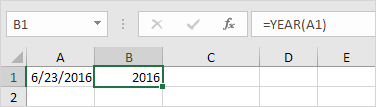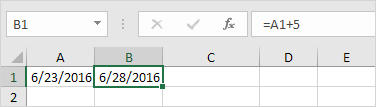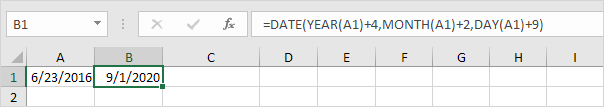# Date & Time Functions

To enter a date in Excel, use the “/” or “-” characters. To enter a time, use the “:” (colon). You can also enter a date and a time in one cell.Note: Dates are in US Format. Months first, Days second. This type of format depends on your windows regional settings. Learn more about Date and Time formats.

### Year, Month, Day

To get the year of a date, use the YEAR function.Note: use the MONTH and DAY function to get the month and day of a date.

### Date Function

1. To add a number of days to a date, use the following simple formula.2. To add a number of years, months and/or days, use the DATE function.Note: the DATE function accepts three arguments: year, month and day. Excel knows that 6 + 2 = 8 = August has 31 days and rolls over to the next month (23 August + 9 days = 1 September).

### Current Date & Time

To get the current date and time, use the NOW function.Note: use the TODAY function to enter today’s date in Excel.

### Hour, Minute, Second

To return the hour, use the HOUR function.Note: use the MINUTE and SECOND function to return the minute and second.

### Time Function

To add a number of hours, minutes and/or seconds, use the TIME function.Note: Excel adds 2 hours, 10 + 1 = 11 minutes and 70 – 60 = 10 seconds.

Previous articleXML in Excel
Next articleArray Formulas in Excel